Github - PeterL1n/BackgroundMattingV2

 - 实时且高分辨率, 在 GPU 上，4K 分辨率(3840x2160) 30fps; HD 分辨率(1920x1080) 60 fps.

 - 基于背景抠图(background matting)，其需要单独捕捉一帧背景图，以用于重建 alpha matte 和前景层.

 - 最关键的挑战是，高分辨率 alpha matte 的计算，在实时处理高分辨率图像的同时，保持发丝级细节.

 - 采用两个神经网络，base 网络计算低分辨率结果; refine 网络对高分辨率图像所选择的图片块( selective patches) 上进一步处理.

 - 两个视频和图像抠图数据集：VideoMatte240K 和 PhotoMatte 13K/85.

## 1. 抠图数据集

### 1.1. 公开数据集

Adobe Deep Image Matting 数据集提供了 269 张人物训练样本和 11 张测试图像，分辨率平均为 1000x1000.

Attention-Guided Hierarchical Structure Aggregation for Image Matting 中362 张人物相关的训练样本和 11 张测试样本，分辨率平均为 1700x2000.

### 1.2. VideoMatte240K

VideoMatte240K 中采集了 484 段高分辨率绿幕(green screen) 视频，并采用色度键抠图(Chroma-key)软件 Adobe After Effects 生成了 240709 张 alpha mattes 和 foregrounds 的视频帧.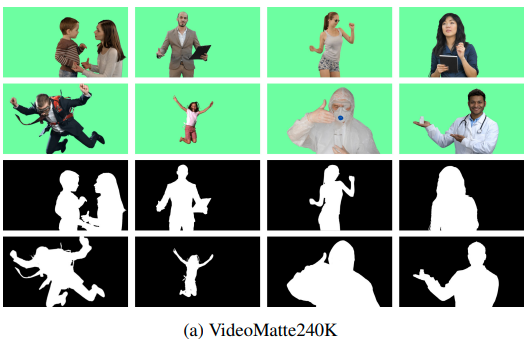### 1.3. PhotoMatte13K/85

PhotoMatte13K/85 中采集了 13665 张图像集合，这些图像是采用摄影棚质量的光照，在绿幕前的相机所拍摄的，并采用色度键抠图算法(Chroma-key) 和手工调整以及错误修正所提取的 mattes.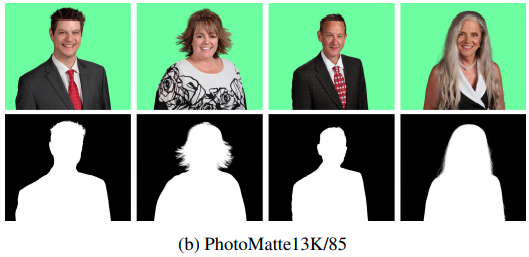## 2. 算法细节

$$I' = \alpha F + (1 - \alpha) B'$$

$$F = max(min( F^{R} + I, 1), 0)$$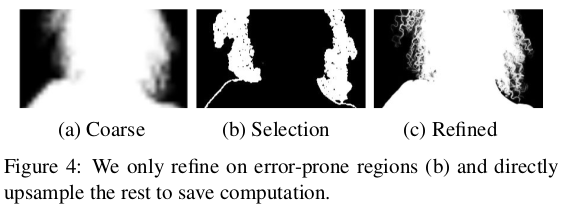- base 网络 $G_{base}$ - 对低分辨率图像进行处理;

 - refinement 网络 $G_{refine}$ - 基于低分辨率预测结果，从原始图像分辨率选择特定图像块进行处理.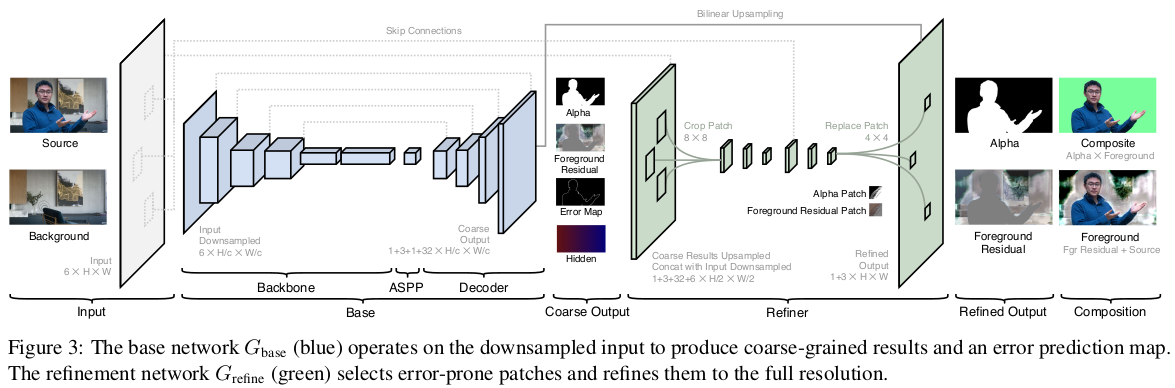$G_{base}$ 采用 $I_c$ 和 $B_c$ 作为输入，并预测输出粗略的 alpha matte $\alpha_c$、foreground residual $F_c^R$、error prediction map $E_c$ 以及 hidden feature $H_c$.

$G_{refine}$ 采用 $H_c$、I 和 B 仅对 $E_c$ 比较大的区域，进一步的精细化 $\alpha_c$ 和 $F_c^R$，并在原始分辨率输出 alpha $\alpha$ 和 $F^R$.

### 2.1. Base 网络

Base 网络受到 DeepLabV3 和 DeepLabV3+ 结构的启发，设计为全卷积的 encoder-decoder 网络，其中主要包括三个模块：Backbone， ASPP，Decoder.

 - Backbone

 - ASPP

ASPP 模块由多个不同 dilation rates 为 3, 6, 9 的 dialted conv filters 组成.

 - Decoder

Decoder 网络的每一步采用双线性上采样(bilinear upsampling)，并通过 skip connection 从 backbone 进行连接，其后接一个 3x3 conv, Batch Normalization 和 ReLU(最后一层例外).

Decoder 网络输出粗略的 alpha matte $\alpha _c$，foreground residual $F_c^R$，error prediction map $E_c$ 和 32 通道 hidden features $H_c$. $H_c$ 包含了有用于 refinement 网络的全局内容信息.

### 2.2. Refinement 网络

Refinement 网络的目标是，降低冗余计算量，并重建高分辨率抠图细节.

Base 网络是对整张图像进行处理，而 Refinement 网络仅对基于 error prediction map $E_c$ 所选择的图像块进行处理.

Refinement 网络是 two-stage 的，分别处理原始分辨率的二分之一和全分辨率.

Refinement 是 two-stage 的，

 - 首先，双线性重采样粗略的输出，如 alpha matte $\alpha_c$、foreground residual $F_c^R$、hidden features $H_c$、输入图像 I和背景图像的分辨率重采样为原始分辨率的 $\frac{1}{2}$，并将这些链接作为特征.

 - 然后，在 $E_4$ 中选择出的 error 位置的周围裁剪出多个 8x8 图像块，并将其分别送入两个 valid padding 的 3x3 conv 层、BN 层和 ReLU 层，以将图像块的分辨率降低为 4x4.

### 2.3. Loss 函数

$$L_{\alpha} = ||\alpha - \alpha^*||_1 + ||\nabla \alpha - \nabla \alpha^*||_1$$

$$F = max(min(F^R + I, 1), 0)$$

$$L_F = ||(\alpha^* > 0) * (F - F^*)||_1$$

$$E^* = |\alpha - \alpha^*|$$

$$L_E = ||E - E^*||_2$$

Base 网络:

$$(\alpha_c, F_c^R, E_c, H_c) = G_{base}(I_c, B_c)$$

$$L_{base} = L_{\alpha_c} + L_{F_C} + L_{E_c}$$

Refinement 网络：

$$(\alpha, F^R) = G_{refine}(\alpha_c, F_{c}^R, E_c, H_c, I, B)$$

$$L_{refine} = L_{\alpha} + L_{F}$$

### 2.4. 训练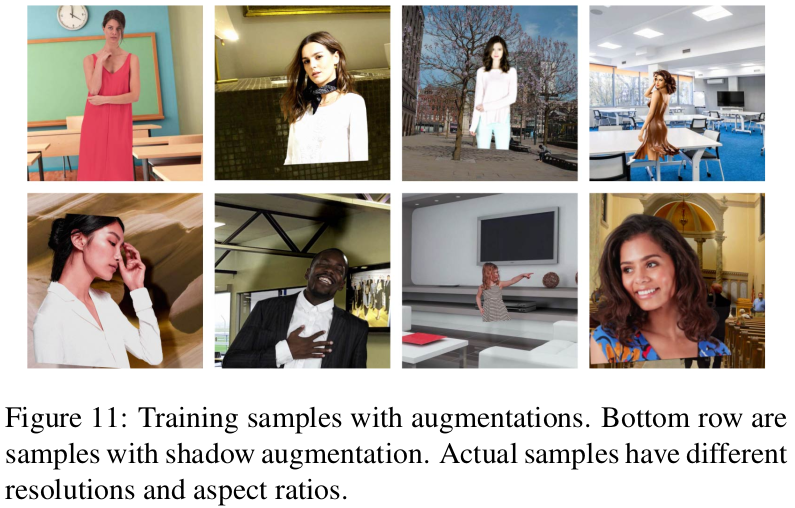- 首先，仅训练 base 网络 $G_{base}$; 在数据集 VideoMatte240K 上，联合训练整个模型 $G_{base}$ 和 $G_{refine}$ ;

 - 然后，在数据集 PhotoMatte 13K 联合训练模型，以提升高分辨率细节.

 - 最后，在公开数据集(646 张训练数据)上联合训练模型.

## 3. Results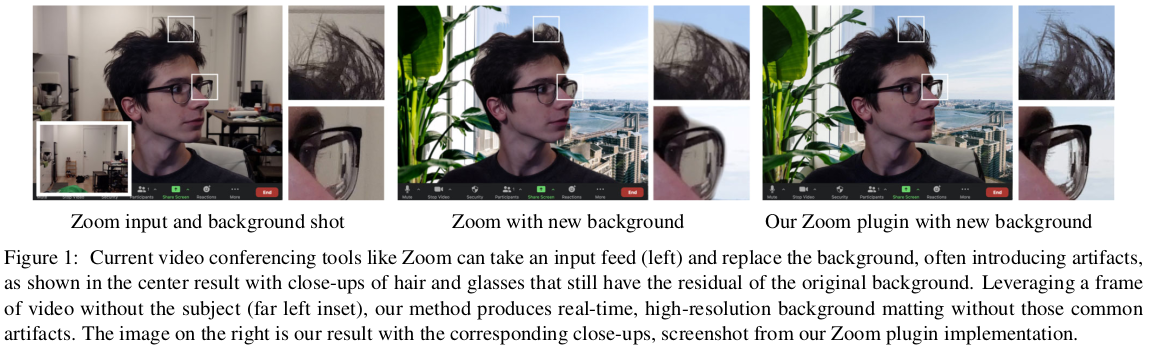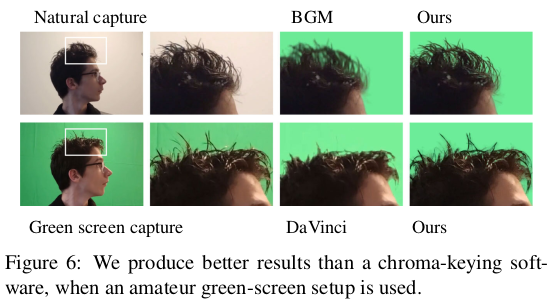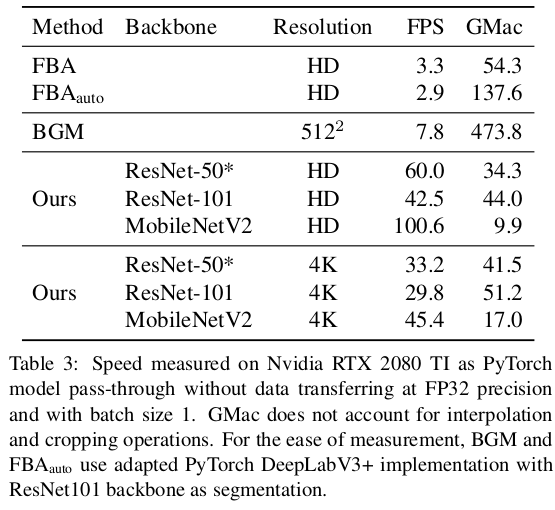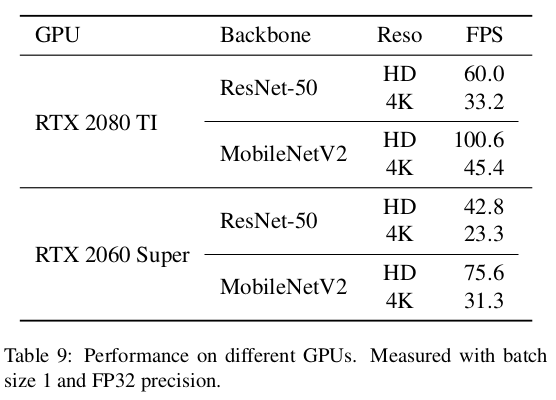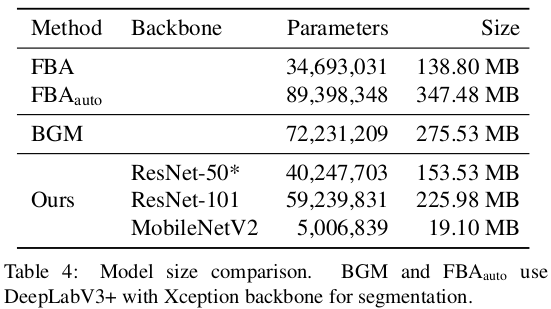Last modification：December 28th, 2020 at 01:41 pm